• 数据处理的主要内容包括数据清洗、数据抽取、数据交换和数据计算等。 数据清洗 数据清洗是数据价值链中最关键的一步。垃圾数据即使是通过最好的分析也可能会产生错误的结果，并造成较的误导。 数据清洗就是...
接着上篇继续。数据获取之后并不能直接分析或使用，因为里面有很多无效的垃圾数据，所以必须要经过处理才可以。数据处理的主要内容包括数据清洗、数据抽取、数据交换和数据计算等。

数据清洗

数据清洗是数据价值链中最关键的一步。垃圾数据即使是通过最好的分析也可能会产生错误的结果，并造成较大的误导。

数据清洗就是处理缺失数据以及清除无意义的信息，如删除原始数据集中的无关数据、重复数据、平滑噪音数据，筛选掉与分析主题无关的数据等等。

重复值的处理

步骤如下：

1 利用DataFrame中的duplicated方法返回一个布尔型的Series，显示是否有重复行。没有显示FALSE,有则从重复的第二行起显示为TRUE

2 在利用DataFrame中的drop_duplicates方法返回一个移除了重复行的DataFrame

duplicated的格式：

duplicated(subset=None, keep='first')

括号中的参数均为可选，不写默认判断全部列

subset用于识别重复的列标签或列标签序号，默认是所有的列标签

keep为first表示除了第一次出现外，其余相同的数据被标记为重复；为last表示除了最后一次外，其余相同的数据被标记为重复；为false表示所有相同的数据都被标记为重复

drop_duplicates的格式：

drop_duplicates()

如果你想指定某个列就在括号里加入列名即可

from pandas import DataFrame
from pandas import Series

#造数据
df=DataFrame({'age':Series([26,85,85]),'name':Series(['xiaoqiang1','xiaoqiang2','xiaoqiang2'])})
df

#判断是否有重复行
df.duplicated()

#移除重复行
df.drop_duplicates()

缺失值的处理

缺失值的处理一般包括两个步骤，分别是缺失数据的识别和缺失数据的处理。

缺失数据的识别

pandas使用浮点值NaN表示浮点和非浮点数组里的缺失数据，并使用isnull和notnull函数来判断缺失情况。

#缺失数据的识别
from pandas import DataFrame

#有缺失数据
df

#识别缺失数据,NaN的就会显示True。notnull函数正好相反
df.isnull()

rz.xlsx的内容如下缺失数据的处理

对于缺失数据的处理有数据补齐、删除对应的行、不处理。这里直接撸代码解释

#接着上面的继续，进行数据的处理
#去除数据中值为空的数据行
newdf=df.dropna()
newdf

#用其他数值代替NaN
newdf2=df.fillna('--')
newdf2

#用前一个数据值代替NaN
newdf3

#用后一个数据值代替NaN
newdf4=df.fillna(method='bfill')
newdf4

#传入一个字典对不同的列填充不同的值
newdf5=df.fillna({'数分':100,'高代':99})
newdf5

#用平均数来代替NaN。会自动计算有NaN两列的数据的平均数
newdf6=df.fillna(df.mean())
newdf6

#还可以使用strip()来去除数据左右的指定字符，这个是python的基础了，这里不做演示了

展开全文• 使用python进行数据处理实例（数据为某公司HR部门关于员工信息的部分摘录，kaggle上面的一次赛题） https://www.kaggle.com/c/kfru-dbm-hr-analytics 该实例是根据其他所给属性预测员工是否会离职，代码实现如下...
 使用python进行数据处理的实例（数据为某公司HR部门关于员工信息的部分摘录，kaggle上面的一次赛题）

https://www.kaggle.com/c/kfru-dbm-hr-analytics

该实例是根据其他所给属性预测员工是否会离职，代码实现如下所示

import pandas as pd
from sklearn.preprocessing import MinMaxScaler,StandardScaler
from sklearn.preprocessing import LabelEncoder,OneHotEncoder
from sklearn.decomposition import PCA

def hr_preprocessing(sl=False,le=False,npr=False,amh=False,tsc=False,wa=False,pl5=False,dp=False,slr=False,lower_id=False,ld_n=1):
#1 清洗数据，根据探索性数据分析出的结果来去掉空值
df=df.dropna(subset=['satisfaction_level','last_evaluation'])
df=df[df['satisfaction_level']<=1][df['salary']!='nme']

#2 得到标注
label=df['left']
df=df.drop('left',axis=1)

#3 特征选择（因为本文的特征本来就不多，暂时不做）

# 4 特征处理（归一化，标准化，降维）
scaler_lst=[sl,le,npr,amh,tsc,wa,pl5]
column_lst = ["satisfaction_level", "last_evaluation", "number_project",
"average_monthly_hours", "time_spend_company", "Work_accident",
"promotion_last_5years"]
for i in range(len(scaler_lst)):
if not scaler_lst[i]:
df[column_lst[i]]=MinMaxScaler().fit_transform(df[column_lst[i]].values.reshape(-1,1)).reshape(1,-1)
else:
df[column_lst[i]]=StandardScaler().fit_transform(df[column_lst[i]].values.reshape(-1,1)).reshape(1,-1)

#针对离散值的处理
scaler_lst=[slr,dp]
column_lst=['salary','department']
for i in range(len(scaler_lst)):
if not scaler_lst[i]:
if column_lst[i]=='salary':
df[column_lst[i]]=[map_salary(s)for s in df['salary'].values]
else:
df[column_lst[i]]=LabelEncoder().fit_transform(df[column_lst[i]])
df[column_lst[i]]=MinMaxScaler().fit_transform(df[column_lst[i]].values.reshape(-1,1)).reshape(1,-1)
else:
df=pd.get_dummies(df,columns=[column_lst[i]])
if lower_id:
return PCA(n_components=ld_n).fit_transform(df.values),label
return df,label

d=dict([('low',0),('medium',1),('high',2)])
def map_salary(s):
return d.get(s,0)

#建模
def hr_modeling_nn(features,label):
from sklearn.model_selection import train_test_split
#sklearn中没有可以一次性将数据划分成验证集、训练集、测试集的包，所以需要进行两步划分
f_v=features.values
l_v=label.values
X_tt,X_validation,Y_tt,Y_validation=train_test_split(f_v,l_v,test_size=0.2)
X_train,X_test,Y_train,Y_test=train_test_split(X_tt,Y_tt,test_size=0.25)
#print(len(X_train),len(X_test),len(X_validation))

#分类
from sklearn.metrics import accuracy_score,recall_score,f1_score
from sklearn.neighbors import NearestNeighbors,KNeighborsClassifier
from sklearn.naive_bayes import GaussianNB,BernoulliNB
from sklearn.tree import DecisionTreeClassifier
from sklearn.svm import SVC
from sklearn.ensemble import RandomForestClassifier
from sklearn.linear_model import LogisticRegression

models=[]
models.append(('KNN',KNeighborsClassifier(n_neighbors=3)))
models.append(('GaussianNB',GaussianNB()))
models.append(('BernoulliNB',BernoulliNB()))
models.append(('DecisionTreeGini',DecisionTreeClassifier()))
models.append(('DecisionTreeEntropy',DecisionTreeClassifier(criterion='entropy')))
models.append(('SVM:',SVC(C=1000)))
models.append(('OriginalRandomForest',RandomForestClassifier()))
models.append(('RandomForest',RandomForestClassifier(n_estimators=11,max_features=None)))
models.append(('LogisticRegression',LogisticRegression(C=1000,tol=1e-10,solver='sag',max_iter=10000)))

for clf_name ,clf in models:
clf.fit(X_train,Y_train)
xy_list=[(X_train,Y_train),(X_validation,Y_validation),(X_test,Y_test)]
for i in range(len(xy_list)):
X_part=xy_list[i]
Y_part=xy_list[i]
Y_pred=clf.predict(X_part)
print(i)
print(clf_name,'-ACC',accuracy_score(Y_part,Y_pred))
print(clf_name,'-REC:',recall_score(Y_part,Y_pred))
print(clf_name,'-F1:',f1_score(Y_pred,Y_part))

#回归
def regr_t(features,label):
print('X',features)
print('Y',label)
from sklearn.linear_model import LinearRegression,Ridge,Lasso
regr=Ridge(alpha=1)
regr.fit(features.values,label.values)
Y_pred=regr.predict(features.values)
print('Coef:',regr.coef_)
from sklearn.metrics import mean_squared_error,mean_absolute_error,r2_score
print('MSE:',mean_squared_error(label.values,Y_pred))
print('MAE:',mean_absolute_error(label.values,Y_pred))
print('R2:',r2_score(label.values,Y_pred))

def main():
features,label=hr_preprocessing()
hr_modeling_nn(features,label)
regr_t(features[['number_project','average_monthly_hours']],features['last_evaluation'])
if __name__=='__main__':
main()




展开全文kaggle 数据处理 python
• 关于数据处理案例有两个，第一个案例是我整理到有道云上的，就直接剪切下来了，下面直接进入正题~ 案例1：快餐数据 案例2：欧洲杯数据 先进行数据探索 data.info() data.describe() 查看...
关于数据处理案例有两个，第一个案例是我整理到有道云上的，就直接剪切下来了，下面直接进入正题~

案例1：快餐数据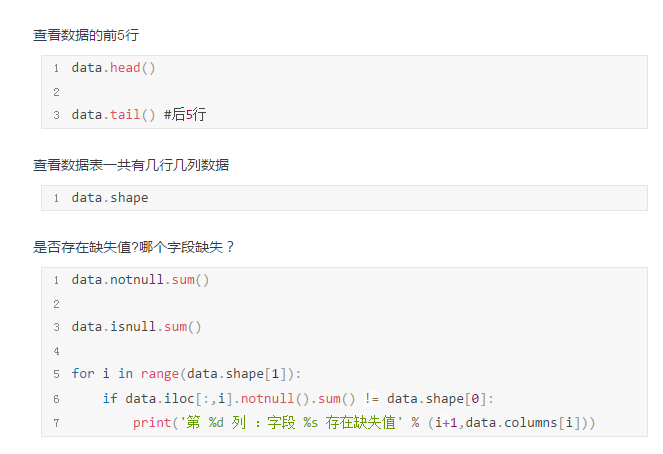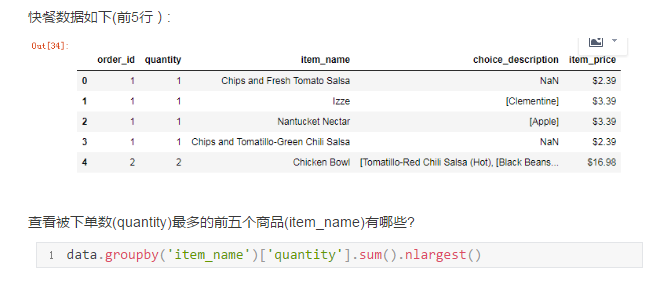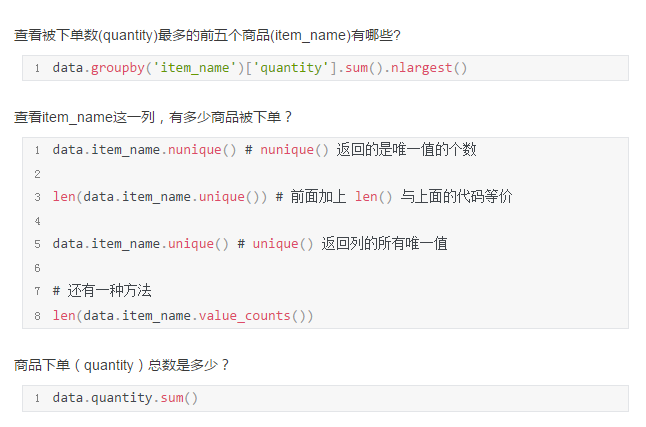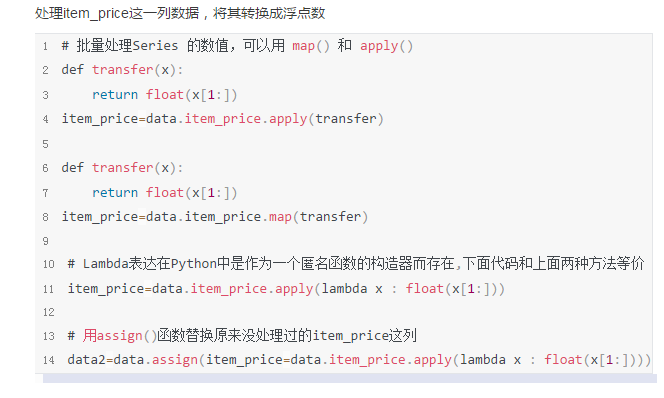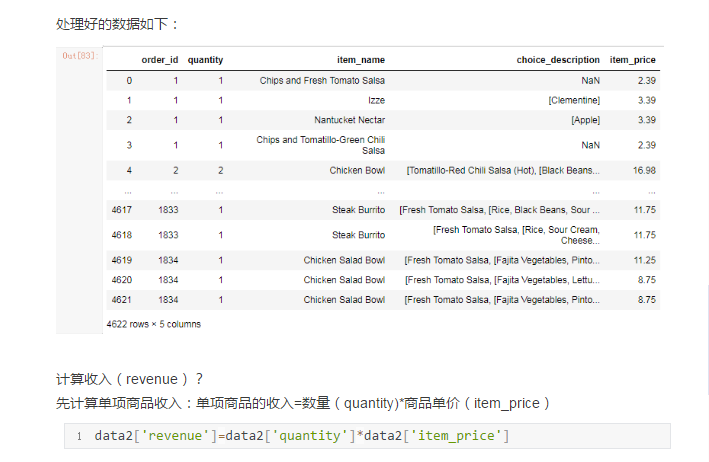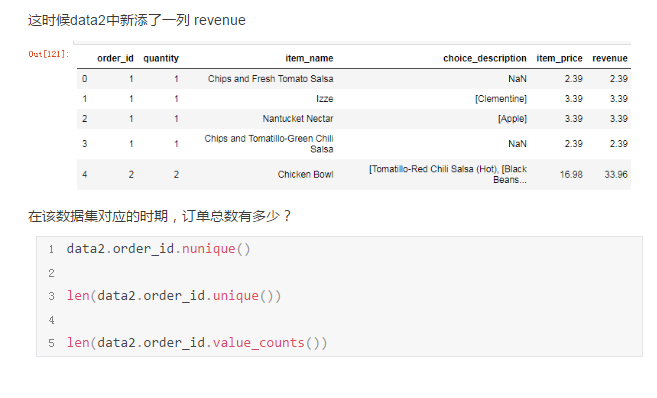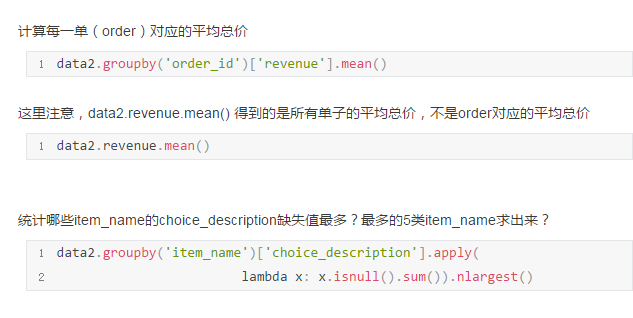案例2：欧洲杯数据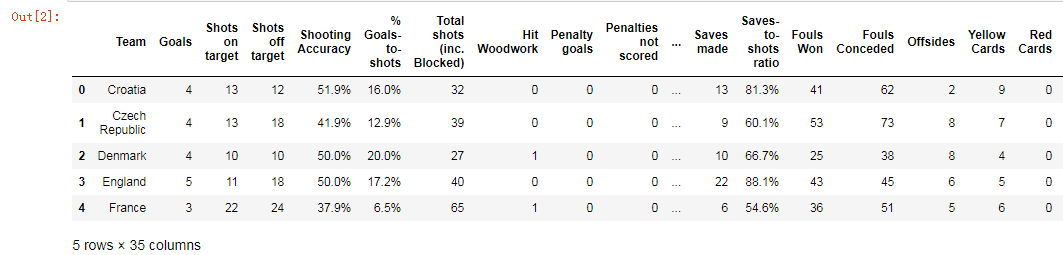先进行数据探索

data.info()

data.describe()


查看数据集是否有缺失值且哪个字段存在缺失值？可以用下面的代码，也可以用前面案例1缺失值那里提到的前两种方法

for i in range(data.shape):
if data.iloc[:,i].notnull().sum() != data.shape:
print('第%d列：字段%s 存在缺失值'%(i+1,data.columns[i]))

代码运行结果是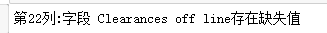对Clearances off line进行缺失值处理

首先查看Clearances off line字段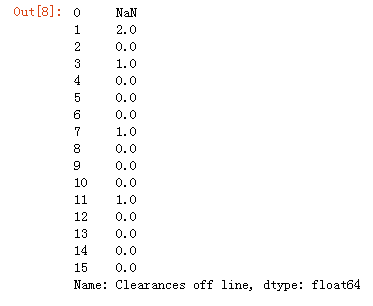统计其数字组成

data['Clearances off line'].value_counts()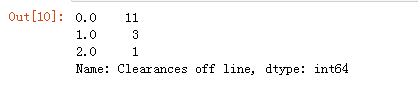从统计结果可以看到，在Clearances off line这个字段中有11个值为0，3个值为1,1个值为2，故考虑采用众数(mode)填充缺失值

mode=data['Clearances off line'].mode()
data['Clearances off line']=data['Clearances off line'].fillna(mode)

描述性统计

统计有多少球队参加了欧洲杯？

data.Team.count()

将数据集中的列Team, Yellow Cards和Red Cards单独存为一个名叫discipline的数据框

discipline=data[['Team','Yellow Cards','Red Cards']]

按照先Red Cards再Yellow Cards进行降序排序

discipline.sort_values(by=['Red Cards','Yellow Cards'])

计算每个球队拿到黄牌的平均值

data['Yellow Cards'].mean()

找出进球数大于6个的球队的数据

data[data['Goals']>6]

对比英格兰(England)、意大利(Italy)和俄罗斯(Russia)的射正率(Shooting Accuracy)

data['Shooting Accuracy'].[data.Team.isin(['England','ltaly','Russia'])]


展开全文• 数据来源百度网盘，提取码：lnqc 二手房数据分析——文件名：lianjia.csv import pandas as pd import numpy as np import seaborn as sns import matplotlib as mpl import matplotlib.pyplot as plt from ...
数据来源百度网盘，提取码：lnqc

二手房数据分析——文件名：lianjia.csv

import pandas as pd
import numpy as np
import seaborn as sns
import matplotlib as mpl
import matplotlib.pyplot as plt
from IPython.display import display
plt.style.use("fivethirtyeight")
sns.set_style({'font.sans-serif':['simhei','Arial']})

# 导入链家二手房数据
lianjia_df.info()
lianjia_df.describe()

#添加新特征房屋均价
df = lianjia_df.copy()
df['PerPrice'] = lianjia_df['Price']/lianjia_df['Size']

#重新摆放列位置
columns = ['Region', 'District', 'Garden', 'Layout', 'Floor', 'Year', 'Size', 'Elevator', 'Direction', 'Renovation', 'PerPrice', 'Price']
df = pd.DataFrame(df, columns = columns)

#重新审视数据集
display(df.head(n=2))

# 对二手房区域分组对比二手房数量和每平米房价
df_house_count = df.groupby('Region')['Price'].count().sort_values(ascending=False).to_frame().reset_index()
df_house_mean = df.groupby('Region')['PerPrice'].mean().sort_values(ascending=False).to_frame().reset_index()

f, [ax1,ax2,ax3] = plt.subplots(3,1,figsize=(30,50))
sns.barplot(x='Region', y='PerPrice', palette="Blues_d", data=df_house_mean, ax=ax1)
ax1.set_title('北京各大区二手房每平米单价对比',fontsize=15)
ax1.set_xlabel('区域')
ax1.set_ylabel('每平米单价')

sns.barplot(x='Region', y='Price', palette="Greens_d", data=df_house_count, ax=ax2)
ax2.set_title('北京各大区二手房数量对比',fontsize=15)
ax2.set_xlabel('区域')
ax2.set_ylabel('数量')

sns.boxplot(x='Region', y='Price', data=df, ax=ax3)
ax3.set_title('北京各大区二手房房屋总价',fontsize=15)
ax3.set_xlabel('区域')
ax3.set_ylabel('房屋总价')

f1, [ax1,ax2] = plt.subplots(1, 2, figsize=(15, 10))
# 建房时间的分布情况
sns.distplot(df['Size'], bins=20, ax=ax1, color='r')
# 建房时间和出售价格的关系
sns.regplot(x='Size', y='Price', data=df, ax=ax2)
plt.show()

df.loc[df['Size']<10]
df.loc[df['Size']>1000]
df = df[(df['Layout']!='叠拼别墅')&(df['Size']<1000)]

# 特征分析
f2, ax1= plt.subplots(figsize=(20,40))
sns.countplot(y='Layout', data=df, ax=ax1)
ax1.set_title('房屋户型',fontsize=15)
ax1.set_xlabel('数量')
ax1.set_ylabel('户型')
plt.show()

df['Renovation'].value_counts()

# 去掉错误数据“南北”，因为爬虫过程中一些信息位置为空，导致“Direction”的特征出现在这里，需要清除或替换
df['Renovation'] = df.loc[(df['Renovation'] != '南北'), 'Renovation']

# 画幅设置
f3, [ax1,ax2,ax3] = plt.subplots(1, 3, figsize=(10, 5))
sns.countplot(df['Renovation'], ax=ax1)
sns.barplot(x='Renovation', y='Price', data=df, ax=ax2)
sns.boxplot(x='Renovation', y='Price', data=df, ax=ax3)
plt.show()

misn = len(df.loc[(df['Elevator'].isnull()), 'Elevator'])
print('Elevator缺失值数量为：'+ str(misn))

# 由于存在个别类型错误，如简装和精装，特征值错位，故需要移除
df['Elevator'] = df.loc[(df['Elevator'] == '有电梯')|(df['Elevator'] == '无电梯'), 'Elevator']

# 填补Elevator缺失值
df.loc[(df['Floor']>6)&(df['Elevator'].isnull()), 'Elevator'] = '有电梯'
df.loc[(df['Floor']<=6)&(df['Elevator'].isnull()), 'Elevator'] = '无电梯'

f5, [ax1,ax2] = plt.subplots(1, 2, figsize=(5, 7))
sns.countplot(df['Elevator'], ax=ax1)
ax1.set_title('有无电梯数量对比',fontsize=3)
ax1.set_xlabel('是否有电梯')
ax1.set_ylabel('数量')
sns.barplot(x='Elevator', y='Price', data=df, ax=ax2)
ax2.set_title('有无电梯房价对比',fontsize=3)
ax2.set_xlabel('是否有电梯')
ax2.set_ylabel('总价')
plt.show()

grid = sns.FacetGrid(df, row='Elevator', col='Renovation', palette='seismic',size=4)
grid.map(plt.scatter, 'Year', 'Price')

f6, ax1= plt.subplots(figsize=(20,5))
sns.countplot(x='Floor', data=df, ax=ax1)
ax1.set_title('房屋户型',fontsize=5)
ax1.set_xlabel('数量')
ax1.set_ylabel('户型')
plt.show()


展开全文• Mysql SQLyog导入导出csv文件SQLyog 导出表中数据存为csv文件1. 选择数据库表 --&gt; 右击属性 --&gt; 备份/导出 --&gt; 导出表数据作为 --&gt; 选择cvs --&gt; 选择下面的“更改” --&gt;...
Mysql SQLyog导入导出csv文件SQLyog 导出表中数据存为csv文件1.    选择数据库表 --> 右击属性 --> 备份/导出 --> 导出表数据作为 --> 选择cvs --> 选择下面的“更改” --> 字段 --> 可变长度--> 字段终止与 -->输入逗号，(这是重点，否则导出的csv文件内容都在一列中，而不是分字段分列)下面两个选项框取消。2.导出csv文件后，使用UE编辑器或者记事本打开，另存为，选择编码为utf-8格式，保存。3.打开csv文件，这样中文为正确的显示，如果不转码保存的话，为中文乱码。SQLyog 将csv文件数据导入mysql表中1.      将数据文件存为csv文件，保存的时候选择逗号(或\t)作为分隔符；2.    选择数据库表 --> 导入 --> 导入本地可使用的CSV数据 --> 从文件导入，选择刚刚的csv文件，导入完成。2.    选择cvs --> 选择下面的“更改” --> 字段 --> 可变长度--> 字段终止与 -->输入逗号，(这是重点，否则导入的csv文件内容都在一列中，而不是分字段分列)下面两个选项框取消。 http://www.cnblogs.com/DswCnblog/p/5970873.html用Python Pandas处理亿级数据在数据分析领域，最热门的莫过于Python和R语言，此前有一篇文章《别老扯什么Hadoop了，你的数据根本不够大》指出：只有在超过5TB数据量的规模下，Hadoop才是一个合理的技术选择。这次拿到近亿条日志数据，千万级数据已经是关系型数据库的查询分析瓶颈，之前使用过Hadoop对大量文本进行分类，这次决定采用Python来处理数据：硬件环境CPU：3.5 GHz Intel Core i7内存：32 GB HDDR 3 1600 MHz硬盘：3 TB Fusion Drive数据分析工具Python：2.7.6Pandas：0.15.0IPython notebook：2.0.0源数据如下表所示： TableSizeDescServiceLogs98,706,832 rows x 14 columns8.77 GB交易日志数据，每个交易会话可以有多条交易ServiceCodes286 rows × 8 columns20 KB交易分类的字典表数据读取启动IPython notebook，加载pylab环境：ipython notebook --pylab=inline1ipython notebook --pylab=inlinePandas提供了IO工具可以将大文件分块读取，测试了一下性能，完整加载9800万条数据也只需要263秒左右，还是相当不错了。import pandas as pd
try:
except StopIteration:
print "Iteration is stopped."123456import pandas as pdreader = pd.read_csv('data/servicelogs', iterator=True)try:    df = reader.get_chunk(100000000)except StopIteration:    print "Iteration is stopped." 1百万条1千万条1亿条ServiceLogs1 s17 s263 s使用不同分块大小来读取再调用 pandas.concat 连接DataFrame，chunkSize设置在1000万条左右速度优化比较明显。loop = True
chunkSize = 100000
chunks = []
while loop:
try:
chunks.append(chunk)
except StopIteration:
loop = False
print "Iteration is stopped."
df = pd.concat(chunks, ignore_index=True)1234567891011loop = TruechunkSize = 100000chunks = []while loop:    try:        chunk = reader.get_chunk(chunkSize)        chunks.append(chunk)    except StopIteration:        loop = False        print "Iteration is stopped."df = pd.concat(chunks, ignore_index=True)下面是统计数据，Read Time是数据读取时间，Total Time是读取和Pandas进行concat操作的时间，根据数据总量来看，对5~50个DataFrame对象进行合并，性能表现比较好。Chunk SizeRead Time (s)Total Time (s)Performance100,000224.418173261.358521 200,000232.076794256.674154 1,000,000213.128481234.934142√ √2,000,000208.410618230.006299√ √ √5,000,000209.460829230.939319√ √ √10,000,000207.082081228.135672√ √ √ √20,000,000209.628596230.775713√ √ √50,000,000222.910643242.405967 100,000,000263.574246263.574246如果使用Spark提供的Python Shell，同样编写Pandas加载数据，时间会短25秒左右，看来Spark对Python的内存使用都有优化。数据清洗Pandas提供了 DataFrame.describe 方法查看数据摘要，包括数据查看（默认共输出首尾60行数据）和行列统计。由于源数据通常包含一些空值甚至空列，会影响数据分析的时间和效率，在预览了数据摘要后，需要对这些无效数据进行处理。首先调用 DataFrame.isnull() 方法查看数据表中哪些为空值，与它相反的方法是 DataFrame.notnull() ，Pandas会将表中所有数据进行null计算，以True/False作为结果进行填充，如下图所示：Pandas的非空计算速度很快，9800万数据也只需要28.7秒。得到初步信息之后，可以对表中空列进行移除操作。尝试了按列名依次计算获取非空列，和 DataFrame.dropna() 两种方式，时间分别为367.0秒和345.3秒，但检查时发现 dropna() 之后所有的行都没有了，查了Pandas手册，原来不加参数的情况下， dropna() 会移除所有包含空值的行。如果只想移除全部为空值的列，需要加上 axis 和 how 两个参数：df.dropna(axis=1, how='all')1df.dropna(axis=1, how='all')共移除了14列中的6列，时间也只消耗了85.9秒。接下来是处理剩余行中的空值，经过测试，在 DataFrame.replace() 中使用空字符串，要比默认的空值NaN节省一些空间；但对整个CSV文件来说，空列只是多存了一个“,”，所以移除的9800万 x 6列也只省下了200M的空间。进一步的数据清洗还是在移除无用数据和合并上。对数据列的丢弃，除无效值和需求规定之外，一些表自身的冗余列也需要在这个环节清理，比如说表中的流水号是某两个字段拼接、类型描述等，通过对这些数据的丢弃，新的数据文件大小为4.73GB，足足减少了4.04G！数据处理使用 DataFrame.dtypes 可以查看每列的数据类型，Pandas默认可以读出int和float64，其它的都处理为object，需要转换格式的一般为日期时间。DataFrame.astype() 方法可对整个DataFrame或某一列进行数据格式转换，支持Python和NumPy的数据类型。df['Name'] = df['Name'].astype(np.datetime64)1df['Name'] = df['Name'].astype(np.datetime64)对数据聚合，我测试了 DataFrame.groupby 和 DataFrame.pivot_table 以及 pandas.merge ，groupby 9800万行 x 3列的时间为99秒，连接表为26秒，生成透视表的速度更快，仅需5秒。df.groupby(['NO','TIME','SVID']).count() # 分组
fullData = pd.merge(df, trancodeData)[['NO','SVID','TIME','CLASS','TYPE']] # 连接
actions = fullData.pivot_table('SVID', columns='TYPE', aggfunc='count') # 透视表123df.groupby(['NO','TIME','SVID']).count() # 分组fullData = pd.merge(df, trancodeData)[['NO','SVID','TIME','CLASS','TYPE']] # 连接actions = fullData.pivot_table('SVID', columns='TYPE', aggfunc='count') # 透视表根据透视表生成的交易/查询比例饼图：将日志时间加入透视表并输出每天的交易/查询比例图：total_actions = fullData.pivot_table('SVID', index='TIME', columns='TYPE', aggfunc='count')
total_actions.plot(subplots=False, figsize=(18,6), kind='area')12total_actions = fullData.pivot_table('SVID', index='TIME', columns='TYPE', aggfunc='count')total_actions.plot(subplots=False, figsize=(18,6), kind='area')除此之外，Pandas提供的DataFrame查询统计功能速度表现也非常优秀，7秒以内就可以查询生成所有类型为交易的数据子表：tranData = fullData[fullData['Type'] == 'Transaction']1tranData = fullData[fullData['Type'] == 'Transaction']该子表的大小为 [10250666 rows x 5 columns]。在此已经完成了数据处理的一些基本场景。实验结果足以说明，在非“>5TB”数据的情况下，Python的表现已经能让擅长使用统计分析语言的数据分析师游刃有余。 
展开全文• Python数据分析案例实战课程大纲：第一课：电力窃漏电用户识别系统案例实战传统的窃漏电分析是通过人工检测来进行的，对人的依赖性太，为了提高窃漏电的判别效率，电力公司决定先根据用户的电表数据进行初步的自动...
• 概述 Excel固然功能强大，也有许多函数实现数据处理功能，但是Excel仍需大量人工操作，虽然能嵌入VB脚本宏，但也...因此，本文就python处理excel数据进行了学习，主要分为python对excel数据处理的常用数据类型以及...
• 对Excel数据进行读取并重新整理至新Excel，并绘制Excel中数据为图表
• （一）案例一介绍 现在有一匹电商产品跟当日销量的数据，如下，总共有上万笔的数据，现在需要统计每个品牌当日的销售量，比如美宝莲今天总共卖出了多少的商品，另外需要统计每个品牌下面的每个子品类当日销售量...
• python数据分析参考案例，在线选房系统代码和数据，网络收集数据分析
• 现在有一个具体的案例是这样的：CST电磁仿真软件得到一些txt数据在origin_data文件夹中。需要其中的一些数据来通过origin软件绘制曲线分析一些问题，而且需要里面的所有数据曲线显示在同一个图形中。如果通过手动将...python
• 问题是这样的， 我是我们学校的寒招队伍的负责人。但是管理的人数实在是太多了。所以在这个地方的，我就想用下脚本去帮助自己进行管理。从效果来看，还是非常方便的。 故事情节： 这次，我和两三个小伙伴们要...python excel
• 本文用到的数据在评论区留下邮箱即可。 本文通过对美国枪杀数据的探索，综合运用python做一些数据的提取和统计python 数据分析 实例
• Python进行Excel数据处理思路实现1. 使用openpyxl2. 使用xlrd和xlwt总结 前两天GF让用Python写个Excel表格处理脚本，在这里记录一下。某公司有个数据统计汇总表格，仅为初表，复制粘贴汇总而来。初表中，存在同一...python excel
• 来自Bitly的usa.gov数据 1、数据说明：该数据是以json格式储存的，该数据集共有十八个维度，我在这里只做两个任务。 a. 对时区进行计数 b. 对windows以及非windows用户进行分组统计，并统计出在相同时区下Windows...python
• 下载地址：网盘下载内容简介······本书采用基于项目的方法，介绍用Python完成数据获取、数据清洗、数据探索、数据呈现、数据规模化和自动化的过程。主要内容包括：Python基础知识，如何从CSV、Excel、XML、...
•    近几年，基于位置服务的应用层出不穷，如GPS车辆导航、打车、外卖、运动等，产生了大量的具有时空信息的轨迹数据，对...本课程讲述了Python对时空数据的处理，以及机器学习方法在空间数据处理上的应用。python工程师 python爬虫 python python教程 python安装
• 最好使用notebook运行.如果直接用使用python运行，只会出现一张图片。python